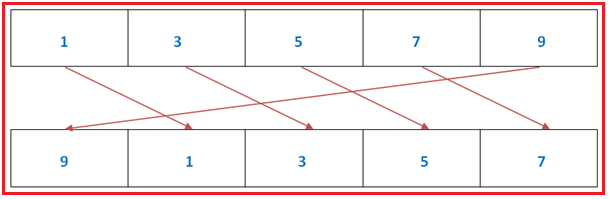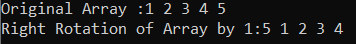# Right Rotation of Array by 1 in C#

## Right Rotation of Array by 1 in C# with Examples

In this article, I am going to discuss the Right Rotation of Array by 1 in C# with Examples. Please read our previous article where we discussed Left Rotation of Array by 1 in C#. The Rotation of the array basically means shifting each and every element to specified positions to the left or right. While writing the program for array rotation we should consider 2 factors:

1. The direction of Rotation – In what direction do we want to perform the rotation. Whether it would be left to right (clockwise) or right to left (anticlockwise).
2. Position of Rotation – At which position rotation will start.
##### Right Rotation of array by 1 in C#In this rotation of the array, each element will be shifted to the right side by position 1. This basically means the value of index 1 will be shifted to index 2(1+1=2), index 2 value will be shifted to index 3(2+1=3), and so on.

Example: From the above fig. We have an array with 5 elements.
Arr = 1,
Arr = 3,
Arr = 5,
Arr = 7,
Arr = 9

So, after performing the right rotation of array 1 the updated values of the array will be
Arr = 9,
Arr = 1,
Arr = 3,
Arr = 5,
Arr = 7

As from the above explanation, it is very clear to us that the changes will be as follows:
Arr = 1 => Aar = 9
Arr = 3 => Arr = 1
Arr = 5 => Arr = 3
Arr = 7 => Arr = 5
Arr = 9 => Arr = 7

Here, we are basically doing what is that except the index last index (n-1 where n is the length of the array) we just need to select the value of indexes one by one and shift that value to the next index.

##### Algorithm to write code

Step1: Create a Temp variable to store the value at index (n-1).
Step2: Sift all the elements of array to right side by position 1 i.e. arr[i] = arr[i-1];
Step3: Store the value of temp at the first 0 index of array i.e. arr = temp;

##### C# Program to perform right rotation of array by position 1.
```using System;
public class LeftRotationOfArray
{
static void Main(string[] args)
{
int[] arr = new int[] { 1, 2, 3, 4, 5 };
Console.Write("Original Array :");
for (int i = 0; i < arr.Length; i++)
{
Console.Write(arr[i] + " ");
}

Console.WriteLine();

LeftRotationOfArray obj = new LeftRotationOfArray();
obj.RigthRotate(arr);
Console.Write("Right Rotation of Array by 1:");

for (int i = 0; i < arr.Length; i++)
{
Console.Write(arr[i] + " ");
}

}

void RigthRotate(int[] arr)
{
int x = arr[(arr.Length - 1)];
for (int i = (arr.Length - 1); i > 0; i--)
{
arr[i] = arr[i - 1];
}

arr = x;
}
}
```
###### Output:In the next article, I am going to discuss how to Rotate an array by K position using Popup and Unshifting Algorithm in C#. Here, in this article, I try to explain the Right Rotation of Array by 1 in C# with Examples and I hope you enjoy this Right Rotation of Array by 1 in C# article.